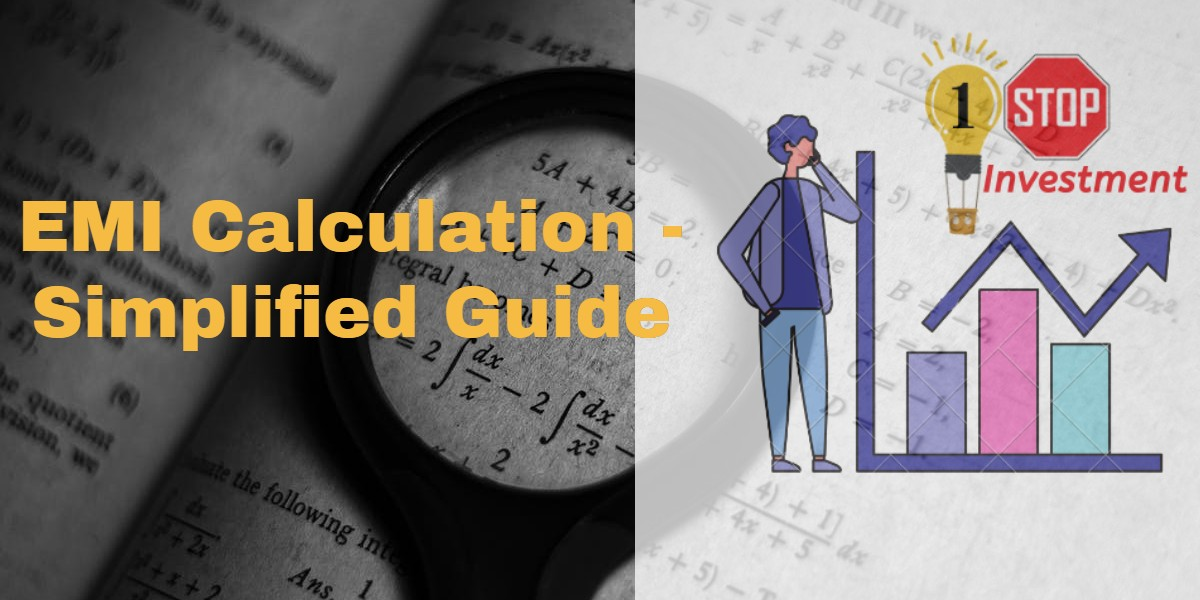## What is EMI ?

EMI – Equated Monthly Instalment !

EMI means repayment of the loan over the fixed period of time.

EMIs are calculated either on Reducing balance method or Flat interest rate method.

EMI have 2 Components – 1.Principal Amount & 2. Interest Amount.

## 1.Flat Interest Rate method

In this method, Interest will be calculated for the loan amount over the loan period.

The EMI payment is same throughout the repayment period.

This is generally applied in Car Loan EMI.For Example, If I am taking a car loan of 5Lakhs at an interest rate of 10% for the period of 3 years.

Interest Calculated will be on Simple Interest formula. (P*N*R)

Interest on 5 Lakhs for 3 years @ 10% = 1.5 Lakhs.

EMI = ( 5 + 1.5 ) / ( 3*12) = 6.5 lakhs / 36 months

EMI = 18055.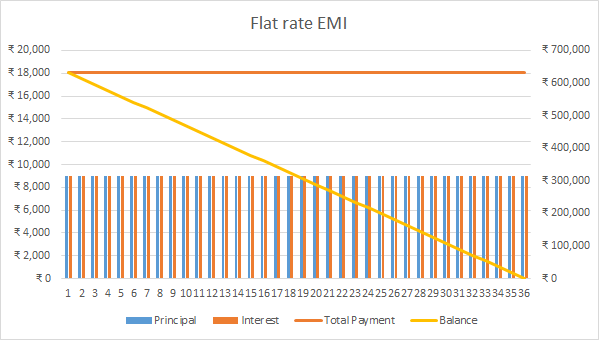## 2.Reducing Balance EMI method

In this method, the interest is calculated on the outstanding balance over the loan amount every month. Hence, EMI to be paid is not the same throughout the repayment period.

This is generally applied in Home Loan EMI.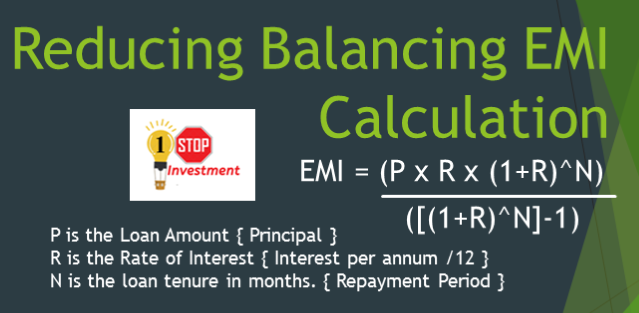If we have take the same above loan of 5 lakhs for 3 years @ 10% interest rate.

P = 5,00,000 , R = 10 / 1200 , N = 36

Calculating using the above formula, EMI = 16,134.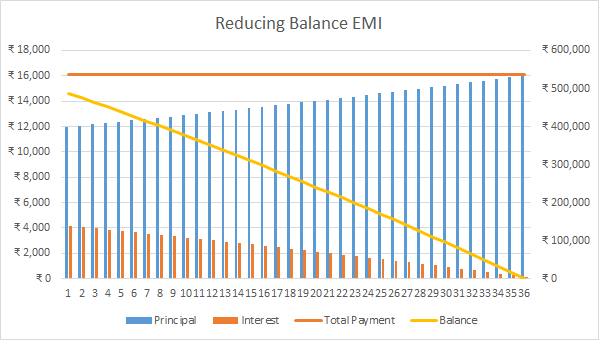By the Reducing balance method, you shall save ₹ 1,922 per month which is ₹ 69,191 in 3 years.

The Reducing balance method is much more economical than the Flat Interest rate loans.

But the problem is Reducing balance EMI Interest rate is higher than the Flat rate loans.

Please Analyze yourself before taking any loans.

Moreover, you can also use the online EMI calculators to calculate the amount you will need to pay every month for the loan taken.

## QUICK EMI GUIDE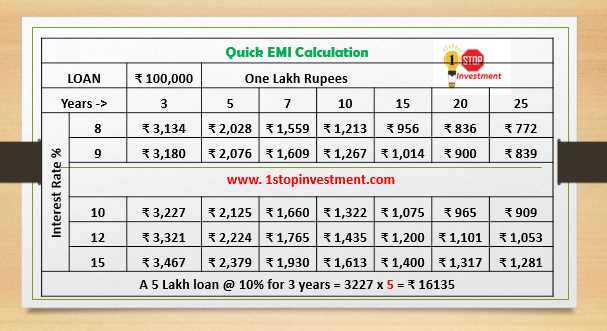Hope you like the quick guide of EMI Calculation.

Share with your friends in Social media if you like.

Share with your friends if you like